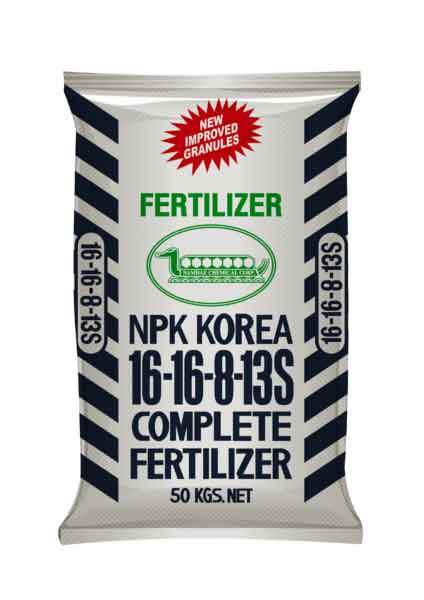Grab a calculator and a copy of the periodic table

(This test has only 10 questions, but may take 15 - 20 mins).

1. Calculate the relative formula mass (Mr) of calcium hydroxide, Ca(OH)2.
• A.   38
• B.   58
• C.   74
• D.   114
2. Which of the following contains the greatest number of moles?
• A.   2.4 g of carbon, C
• B.   2.3 g of sodium, Na
• C.   2.4 g of Magnesium, Mg
• D.   3.9 g of potassium, K
3. A student required 0.15 moles of magnesium oxide, MgO, for a reaction. What mass of MgO should she weigh out? [Mr of MgO is 40]
• A.   0.00375 g
• B.   0.0060 g
• C    6.0 g
• D.   267 g
4. Which of the following statements about chemical reactions is true?

The total mass of the reactants is ...

• A.  .. equal to the total mass of the products in all chemical reactions
• B.   ..less than the total mass of the products in a precipitation reaction in a closed flask
• C.   ..is greater than the total mass of the products in a precipitation reaction in a closed flask
• D.  .. is greater than the total mass of the products in a reaction producing a gas in an open flask
5. 60.0g of calcium carbonate decomposed on heating to form calcium oxide and carbon dioxide.   The equation for the reaction is:

CaCO3  CaO + CO2

What is the maximum mass of calcium oxide which could be formed in this reaction?
[Mr values: CaCO3 = 100; CaO = 56; CO2 = 44]

• A.   26.4 g
• B.   30.0 g
• C.   33.6 g
• D.   56.0 g
6. A thermometer has a scale mark at every 0.5°C. What is the best estimate of the uncertainty of any temperature readings made using this thermometer?
• A.   ±0.1°C
• B.   ±0.25°C
• C.   ±0.5°C
• D.   ±1°C
7. A student recorded the time taken for a piece of magnesium to disappear in acid. For reliability, he repeated his experiment 5 times and obtained the following results:

42.0s, 44.0s, 43.5s, 43.0s, 42.5s
What is the mean value and best estimate of the uncertainty for this set of readings?

• A.   43.0 ± 0.25 s
• B.   43.0 ± 0.5 s
• C.   43.0 ± 1.0 s
• D.   43.5 ± 0.1 s
8. 1.38 g of sodium was burned in oxygen. An oxide of mass 2.34 g was formed.

Calculate the moles of sodium and oxygen atoms in the final product.
[Ar of Na = 23; Ar of O = 16]

 Moles of sodium atoms Moles of oxygen atoms A 0.06 0.146 B 0.06 0.06 C 0.102 0.06 D 0.102 0.146

Q9-10: 34.0 kg of ammonia, NH3 is reacted with excess nitric acid, HNO3, to produce ammonium nitrate fertilizer. The equation for the reaction is:

NH3  + HNO3  NH4NO39. Calculate the number of moles of ammonia, NH3, used in this reaction.

[Relative atomic masses H = 1; N = 14, O = 16]

• A.   2.27
• B.   2.00
• C.   2267
• D.   2000
10. Calculate the maximum mass, in kg, of ammonium nitrate formed [Mr of NH4NO3 is 80]
• A.   181.6 kg
• B.   160 kg
• C.   181,600 kg
• D.   160,000 kg